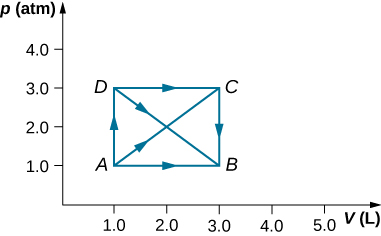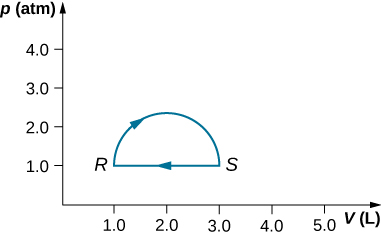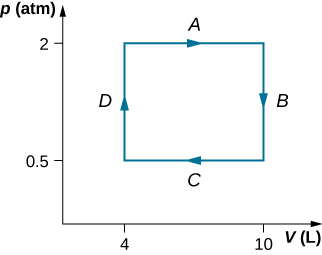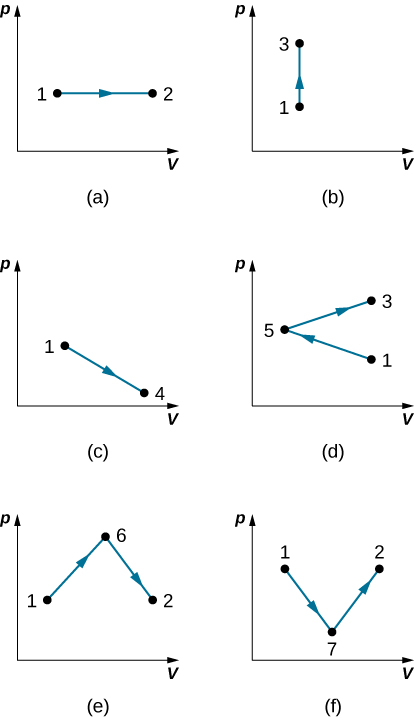# 3.2 Work, heat, and internal energy  (Page 4/6)

 Page 4 / 6

## Conceptual questions

Is it possible to determine whether a change in internal energy is caused by heat transferred, by work performed, or by a combination of the two?

When a liquid is vaporized, its change in internal energy is not equal to the heat added. Why?

Some of the energy goes into changing the phase of the liquid to gas.

Why does a bicycle pump feel warm as you inflate your tire?

Is it possible for the temperature of a system to remain constant when heat flows into or out of it? If so, give examples.

Yes, as long as the work done equals the heat added there will be no change in internal energy and thereby no change in temperature. When water freezes or when ice melts while removing or adding heat, respectively, the temperature remains constant.

## Problems

A gas at a pressure of 2.00 atm undergoes a quasi-static isobaric expansion from 3.00 to 5.00 L. How much work is done by the gas?

It takes 500 J of work to compress quasi-statically 0.50 mol of an ideal gas to one-fifth its original volume. Calculate the temperature of the gas, assuming it remains constant during the compression.

74 K

It is found that, when a dilute gas expands quasi-statically from 0.50 to 4.0 L, it does 250 J of work. Assuming that the gas temperature remains constant at 300 K, how many moles of gas are present?

In a quasi-static isobaric expansion, 500 J of work are done by the gas. If the gas pressure is 0.80 atm, what is the fractional increase in the volume of the gas, assuming it was originally at 20.0 L?

1.4 times

When a gas undergoes a quasi-static isobaric change in volume from 10.0 to 2.0 L, 15 J of work from an external source are required. What is the pressure of the gas?

An ideal gas expands quasi-statically and isothermally from a state with pressure p and volume V to a state with volume 4V. Show that the work done by the gas in the expansion is pV(ln 4).

pVln(4)

As shown below, calculate the work done by the gas in the quasi-static processes represented by the paths (a) AB; (b) ADB; (c) ACB; and (d) ADCB.(a) Calculate the work done by the gas along the closed path shown below. The curved section between R and S is semicircular. (b) If the process is carried out in the opposite direction, what is the work done by the gas?a. 160 J; b. –160 J

An ideal gas expands quasi-statically to three times its original volume. Which process requires more work from the gas, an isothermal process or an isobaric one? Determine the ratio of the work done in these processes.

A dilute gas at a pressure of 2.0 atm and a volume of 4.0 L is taken through the following quasi-static steps: (a) an isobaric expansion to a volume of 10.0 L, (b) an isochoric change to a pressure of 0.50 atm, (c) an isobaric compression to a volume of 4.0 L, and (d) an isochoric change to a pressure of 2.0 atm. Show these steps on a pV diagram and determine from your graph the net work done by the gas.$W=900\phantom{\rule{0.2em}{0ex}}\text{J}$

What is the average mechanical energy of the atoms of an ideal monatomic gas at 300 K?

What is the internal energy of 6.00 mol of an ideal monatomic gas at $200\phantom{\rule{0.2em}{0ex}}\text{°}\text{C}$ ?

$3.53\phantom{\rule{0.2em}{0ex}}×\phantom{\rule{0.2em}{0ex}}{10}^{4}\phantom{\rule{0.2em}{0ex}}\text{J}$

Calculate the internal energy of 15 mg of helium at a temperature of $0\phantom{\rule{0.2em}{0ex}}\text{°}\text{C}.$

Two monatomic ideal gases A and B are at the same temperature. If 1.0 g of gas A has the same internal energy as 0.10 g of gas B, what are (a) the ratio of the number of moles of each gas and (b) the ration of the atomic masses of the two gases?

a. 1:1; b. 10:1

The van der Waals coefficients for oxygen are $a=0.138\phantom{\rule{0.2em}{0ex}}\text{J}·{\text{m}}^{3}\text{/}{\text{mol}}^{2}$ and $b=3.18\phantom{\rule{0.2em}{0ex}}×\phantom{\rule{0.2em}{0ex}}{10}^{-5}{\phantom{\rule{0.2em}{0ex}}\text{m}}^{3}\text{/}\text{mol}$ . Use these values to draw a van der Waals isotherm of oxygen at 100 K. On the same graph, draw isotherms of one mole of an ideal gas.

Find the work done in the quasi-static processes shown below. The states are given as (p, V) values for the points in the pV plane: 1 (3 atm, 4 L), 2 (3 atm, 6 L), 3 (5 atm, 4 L), 4 (2 atm, 6 L), 5 (4 atm, 2 L), 6 (5 atm, 5 L), and 7 (2 atm, 5 L).a. 600 J; b. 0; c. 500 J; d. 200 J; e. 800 J; f. 500 J

what is principle of superposition
what are questions that are likely to come out during exam
what is electricity
watt is electricity.
electricity ka full definition with formula
Jyoti
If a point charge is released from rest in a uniform electric field will it follow a field line? Will it do so if the electric field is not uniform?
Maxwell's stress tensor is
Yes
doris
neither vector nor scalar
Anil
if 6.0×10^13 electrons are placed on a metal sphere of charge 9.0micro Coulombs, what is the net charge on the sphere
18.51micro Coulombs
ASHOK
Is it possible to find the magnetic field of a circular loop at the centre by using ampere's law?
Is it possible to find the magnetic field of a circular loop at it's centre?
yes
Brother
The density of a gas of relative molecular mass 28 at a certain temperature is 0.90 K kgmcube.The root mean square speed of the gas molecules at that temperature is 602ms.Assuming that the rate of diffusion of a gas in inversely proportional to the square root of its density,calculate the density of
A hot liquid at 80degree Celsius is added to 600g of the same liquid originally at 10 degree Celsius. when the mixture reaches 30 degree Celsius, what will be the total mass of the liquid?
Under which topic
doris
what is electrostatics
Study of charges which are at rest
himanshu
Explain Kinematics
Two equal positive charges are repelling each other. The force on the charge on the left is 3.0 Newtons. Using your notes on Coulomb's law, and the forces acting on each of the charges, what is the force on the charge on the right?
Using the same two positive charges, the left positive charge is increased so that its charge is 4 times LARGER than the charge on the right. Using your notes on Coulomb's law and changes to the charge, once the charge is increased, what is the new force of repulsion between the two positive charges?
Nya
A mass 'm' is attached to a spring oscillates every 5 second. If the mass is increased by a 5 kg, the period increases by 3 second. Find its initial mass 'm'
a hot water tank containing 50,000g of water is heated by an electric immersion heater rated at 3kilowatt,240volt, calculate the current
what is charge
product of current and time
JaffarBy Brooke DelaneyBy OpenStaxBy Jonathan LongBy OpenStaxByBy Anonymous UserBy Madison ChristianBy Vongkol HENGBy Jill ZerressenBy OpenStax